# Quantitative Aptitude Quiz For SBI, IBPS Prelims 2021- 6th March

Directions (1-5): Solve the given quadratic equations and mark the correct option based on your answer—
(a) x > y
(b) x ≥ y
(c) x < y
(d) x ≤ y
(e) x = y or no relation can be established between x and y.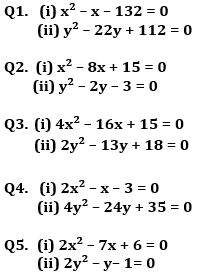Directions (6-10): In each of these questions, two equations (I) and (II) are given. You have to solve both the equations and give answer
(a) if x>y
(b) if x≥y
(c) if x<y
(d)if x ≤y
(e) if x = y or no relation can be established between x and y.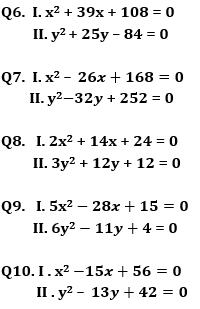Directions (11-15): In the following questions, two equations numbered I and II are given. You have to solve both the equations and give answers among the following options.

(a) if x>y
(b) if x≥y
(c) if x<y
(d) if x ≤y
(e) if x=y or the relationship cannot be established.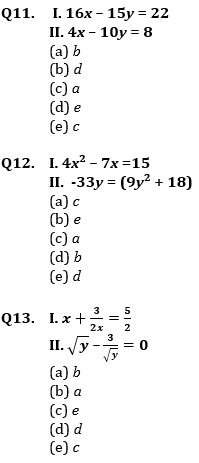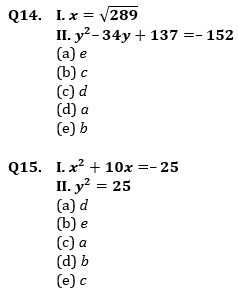Practice More Questions of Quantitative Aptitude for Competitive Exams:

###### Study Plan for IBPS and SBI Exams 2021

Solutions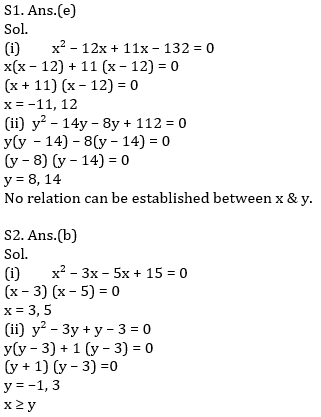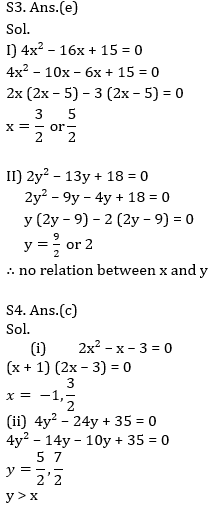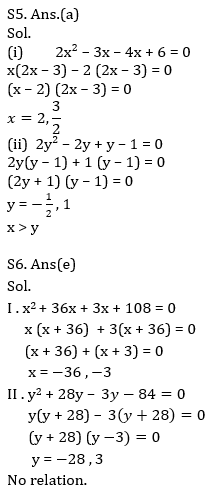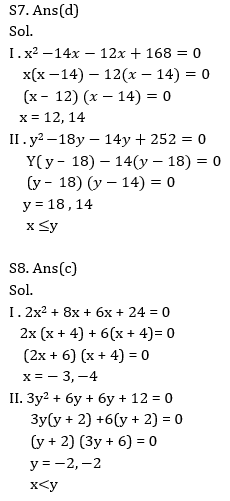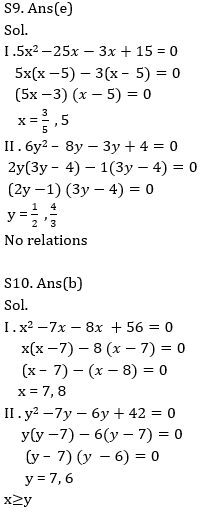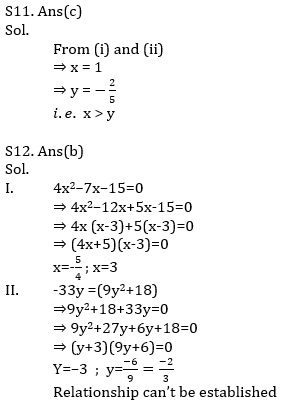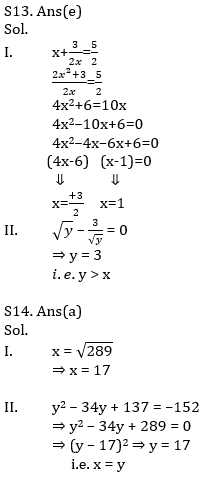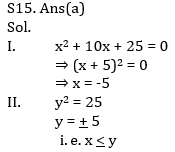Practice with Online Test Series for SBI and IBPS Prelims 2021:

Click Here to Register for Bank Exams 2020 Preparation Material×

## Download success!

Thanks for downloading the guide. For similar guides, free study material, quizzes, videos and job alerts you can download the Adda247 app from play store.

Thank You, Your details have been submitted we will get back to you.
×
Login
OR

Forgot Password?

×
Sign Up
ORForgot Password
Enter the email address associated with your account, and we'll email you an OTP to verify it's you.Reset Password
Please enter the OTP sent to
/6

×
CHANGE PASSWORD